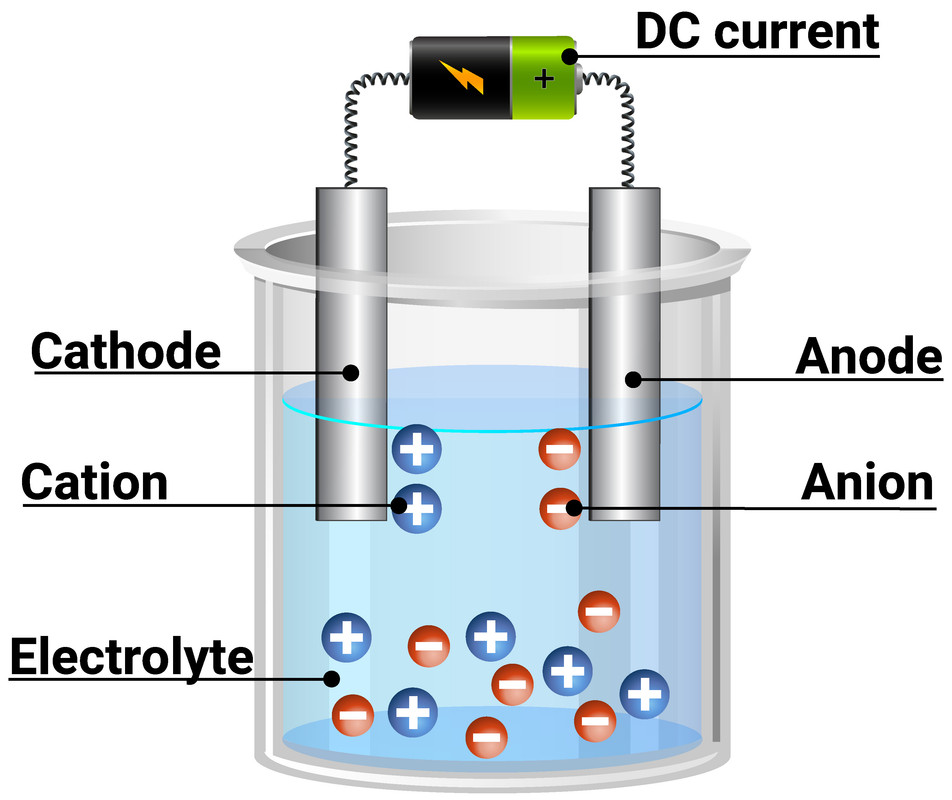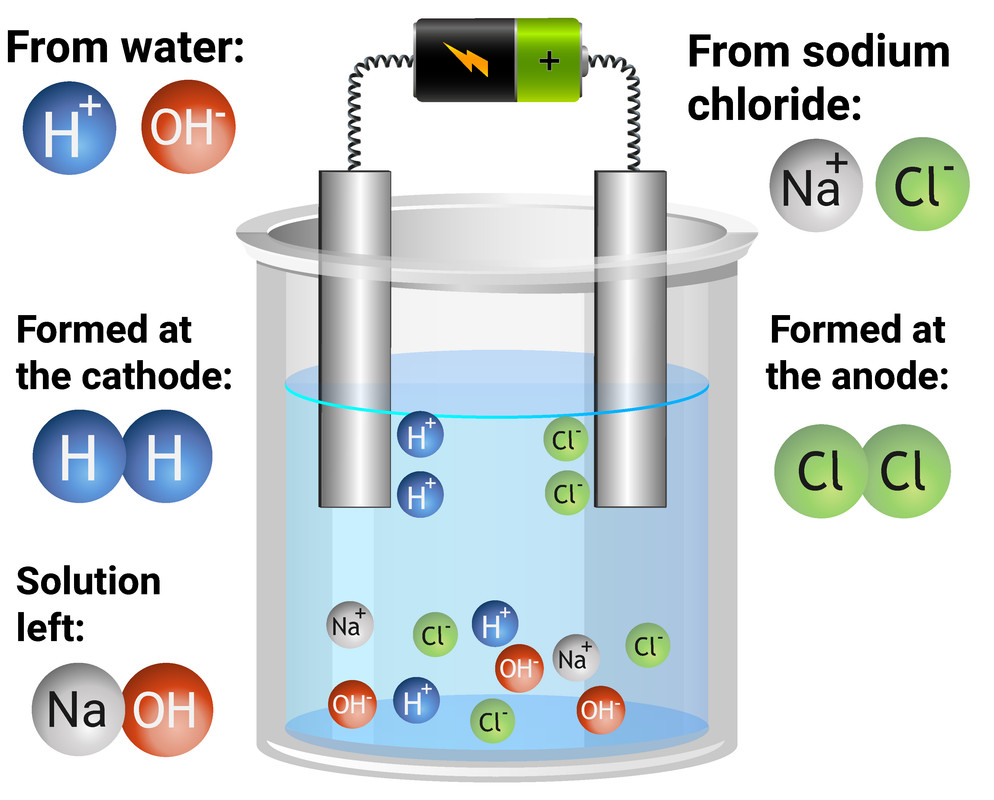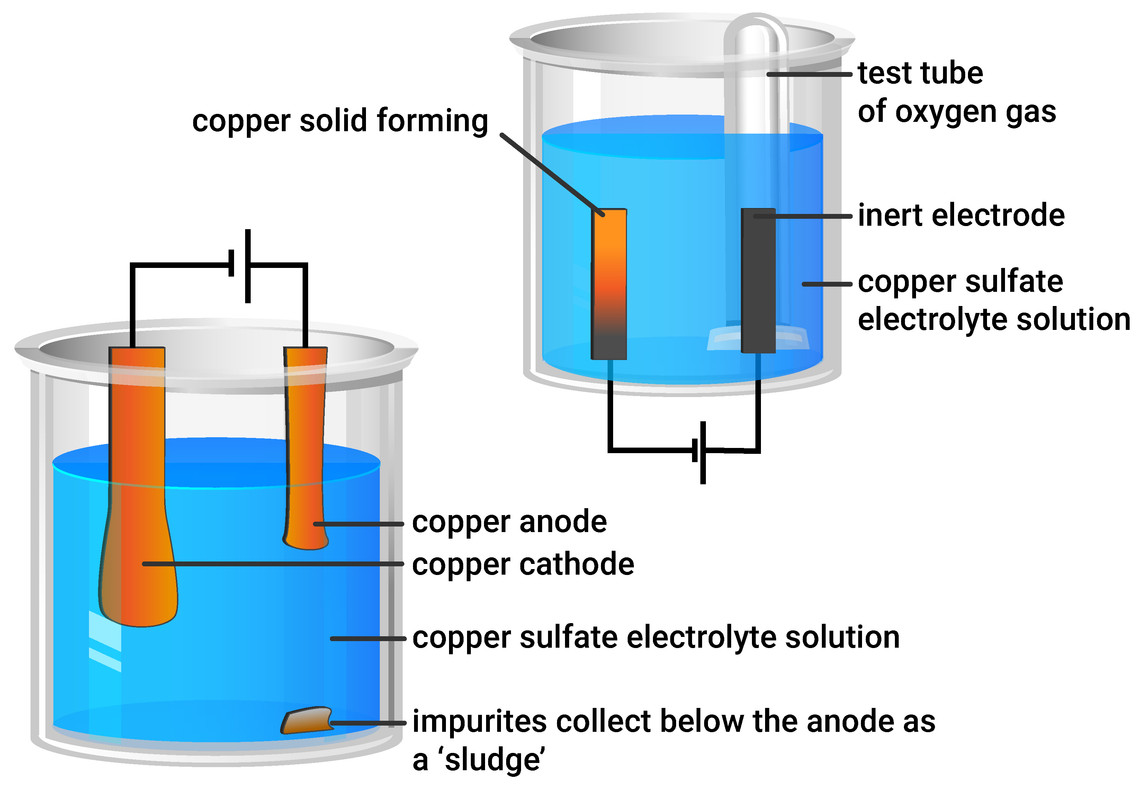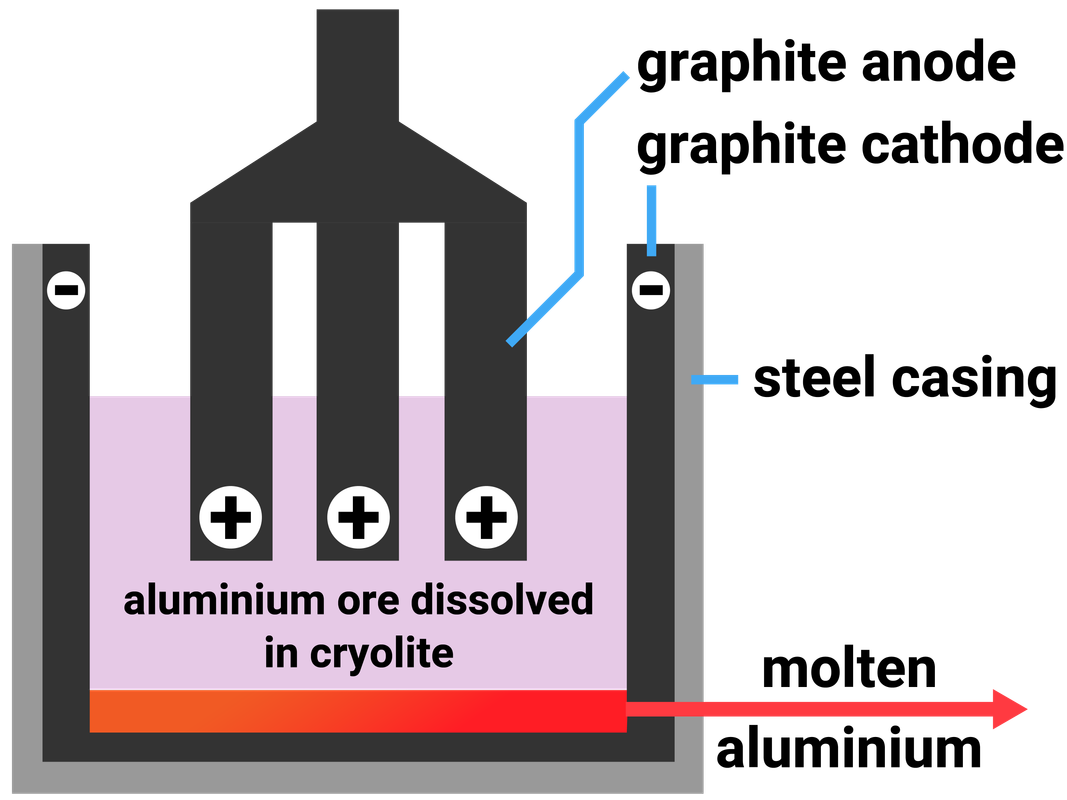# Electrolysis

### Electrolysis

Electrolysis is the process of passing electrical current (direct current) through a solution or molten ionic compound, to decompose electrolytes. This separates the ions, and elements will be discharged at the electrodesInert electrodes should be used, otherwise they will react with the solution/products.

Electrolysis can only be done using ionic compounds, as it requires free ions to move. This is why it can only be done if in solution, or liquid (molten) state. If electrolysis is completed with a solution, then hydrogen (H+) and hydroxide (OH-) ions will also be present, as water will also dissociate.

During electrolysis:

• positively charged ions (cations) migrate to the negatively charged electrode (cathode)
• negatively charged ions (anions) migrate to the positively charged electrode (anode)

### Rules of electrolysis

• at the cathode (negative electrode) - the least reactive element (metal or hydrogen) will be discharged
• at the anode (positive electrode) - in order of preference:
1. halide ions (F⁻, Cl⁻, Br⁻ etc.) will be discharged as halogen gases
2. hydroxide ions (OH⁻) - given off as oxygen gas
3. any other negative ions present### Electrolysis of Solution

You need to be able to explain the formation of products during specific electrolysis experiments, when inert electrodes are used. In the table below, we can see which products are formed. If you are unsure why the following discharges happen, look again above at the rules of electrolysis.

Electrolyte At the anode (+) At the cathode (-)
copper chloride (aq)
(CuCl2)
chlorine gas copper metal
sodium chloride (aq)
(NaCl)
chlorine gas
hydrogen gas
sodium sulfate (aq)
(Na2SO4)
oxygen gas hydrogen gas
water acidified with sulfuric acid
(H+/H2O)
oxygen gas hydrogen gas
(PbBr2)### Purifying copper

Copper can be purified by electrolysis. Electricity is passed through solutions containing copper compounds, such as copper sulfate. In this process, the anode would be made from impure copper and the cathode made from pure copper.

Copper ions are positive (Cu2+), so move to the negative electrode (cathode). During electrolysis, the anode loses mass as copper dissolves (as the ions are moving to the cathode), and the cathode gains mass as copper is deposited.

Impurities will form under the impure copper anode, in the form of a 'sludge'. This shouldn't be immediately thrown away, as it may contain valuable metals like silver.

### Electrolysis of copper sulfate with graphite electrodes

Using inert electrodes (such as graphite electrodes) will still produce copper deposits at the cathode. However, rather than the anode mass decreasing (like it does in copper purification) we will produce oxygen gas. This can be tested for using a glowing splint, and if it relights - then oxygen is present.### Extracting Metals

The least reactive metals are found in their native state in the Earth's crust. This means they are found as uncombined elements.

Any metal more reactive than hydrogen, but less reactive than carbon, can be extracted by adding carbon to displace the metal from its oxide (or other compounds). This is known as reduction.

The most reactive metals have to be extracted using electrolysis, as carbon is not reactive enough to displace the metals. This is rather costly, and uses a lot of electricity.

### Extracting Aluminium

Aluminium oxide is insoluble in water, so it must be molten to act as an electrolyte. A lot of energy must be transferred to break aluminium's ionic bonds, which is expensive - so to reduce costs, powdered aluminium oxide is dissolved in molten cryolite. This melts at a lower temperature than aluminium oxide and helps reduce costs.

Higher Tier

Half equation at the cathode:

Al3+ + 3e- → Al

Half equation at the anode:

2O2- → O2 + 4e-### Half Equations

Higher Tier

A half equation shows what happens at each electrode during electrolysis, in terms of the electron transfer. It shows what happens when ions gain electrons (reduction) or lose electrons (oxidation). In half equations:

• electrons are shown as e-
• the numbers of atoms of each element must be the same on both sides (like in a balanced equation)
• the total charge on each side needs to be the same

### At the cathode

Positive ions (cations) will gather at the cathode, and gain electrons. You will either need to write a half equation for a metal, or hydrogen gaining electrons.

Group one metals, and hydrogen, only have a +1 charge, and so only need to gain one electron, but notice how because hydrogen gas is made of two hydrogen atoms, we must start with 2 hydrogen ions:

Na+(aq) + e- → Na(s)
K+(aq) + e- → K(s)
2H+(aq) + 2e- → H2(g)
Cu2+(aq) + 2e- → Cu(s)

### At the anode

Negative ions (anions) will gather at the anode, and lose electrons. You will either need to write a half equation for a halide, oxygen, hydroxide or a metal.

2Cl-(aq) - 2e- → Cl2(g)
2O2-(aq) - 4e- → O2(g)
4OH-(aq) - 4e- → 2H2O(l) + O2(g)
Cu(s) - 2e- → Cu2+(aq)